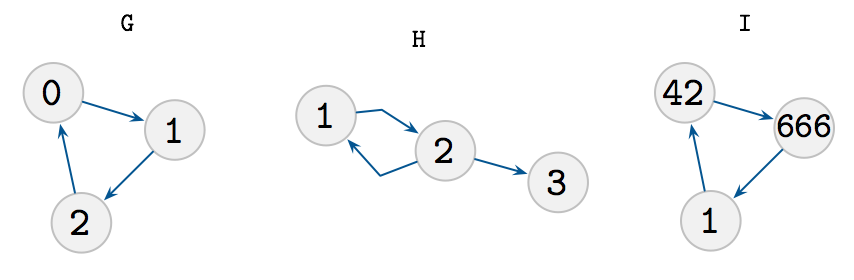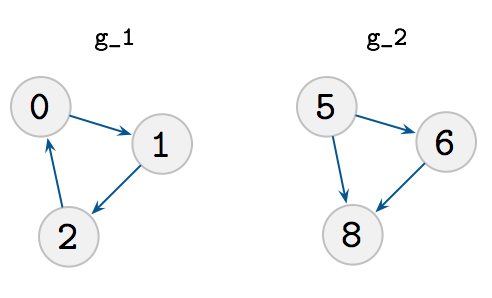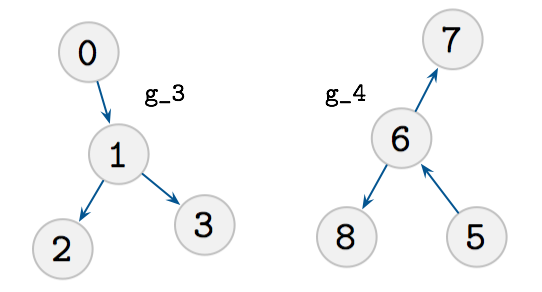## graph-isomorphisms

0.1.0 • Public • Published

# Are two graphs isomorphic?

This is a small JS library that can check how many isomorphisms exists between two graphs.

It is implement using unification (based on datascript) and runtime is exponential in the number of nodes. In other words, you can only check very small graphs.

### Examples### Note on runtime complexity.

Graph Isomorphism is a famous problem in computer science, on which some recent progress has been made by László Babai, giving an algorithm that runs in "quasi-polynomial-time", e.g. efficient. We don't implement this algorithm, obviously.

https://www.quantamagazine.org/20151214-graph-isomorphism-algorithm/

## API

A graph is specified as an edge list, an array of pairs of numbers specifying a directed edge from the first to the second node.

For example, take these two graphs.You can now ask if these two are isomorphic (they shouldn't be).Actually, if they are isomorphic, you will get proofs: a mapping between the edges (negative numbers) and nodes.

Notice the negative zero, LOL floating point numbers

That's it.

## Keywords

### Repository

github.com/wires/graph-isomorphisms

2

0.1.0